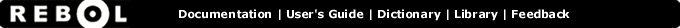# < - Function Summary

## Summary:

Returns TRUE if the first value is less than the second value.

< value1 value2

## Arguments:

value1 - The value1 argument.

value2 - The value2 argument.

## Description:

Returns FALSE for all other values. An error will occur if the values are not of the same datatype. For string-based datatypes, the sorting order is used for comparison with character casing ignored (uppercase = lowercase).

 ``` print "abc" < "abcd" true```

 ``` print 12-June-1999 < 10-June-1999 false```

 ``` print 1.2.3.4 < 4.3.2.1 true```

 ``` print 1:30 < 2:00 true```

## Related:

<= - Returns TRUE if the first value is less than or equal to the second value.
<> - Returns TRUE if the values are not equal.
= - Returns TRUE if the values are equal.
> - Returns TRUE if the first value is greater than the second value.
>= - Returns TRUE if the first value is greater than or equal to the second value.
lesser? - Returns TRUE if the first value is less than the second value.
max - Returns the greater of the two values.
min - Returns the lesser of the two values.